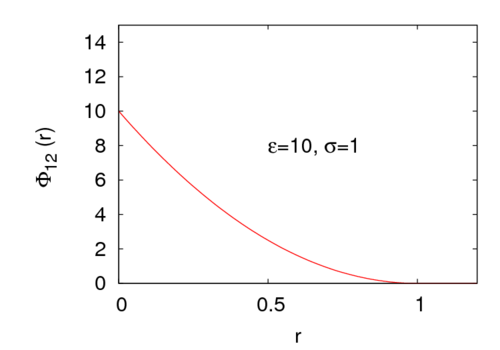# Hertzian sphere model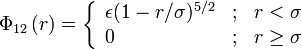$\Phi_{12}\left( r \right) = \left\{ \begin{array}{lll} \epsilon (1-r/\sigma)^{5/2} & ; & r < \sigma \\ 0 & ; & r \geq \sigma \end{array} \right.$
where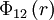$\Phi_{12}\left( r \right)$ is the intermolecular pair potential,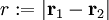$r := |\mathbf{r}_1 - \mathbf{r}_2|$ is the distance between site 1 and site 2.$\sigma$ represents a length scale and$\epsilon$ an energy. In the limit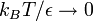$k_BT/\epsilon \rightarrow 0$ this potential becomes the hard sphere model. For example, for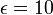$\epsilon=10$ the potential looks like